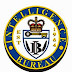## 728x90 AdSpace

Monday, February 23, 2015

# IB ACIO Exam 2015 Solved Question Paper- Part 2

16) Where was Bhaskara, also known as Bhaskaracharya or Bhaskara II, an Indian mathematician & astronomer, was born?
Ans. (3) Bijapur
17)  The BrahMos, a short range supersonic cruise missile, is a joint venture between which foreign country and Indian agency?
Ans. (4) Russia & DRDO

18) If the sum of 60% of a fractional number and the number’s square root is 5 greater than one fifth of the number, then the number is?
Ans. (1) 6.25

19) If ‘Head’ appears consecutively in the first three tosses of a fair/unbiased coin, what is the probability of ‘Head’ appearing in the fourth toss also?
Ans. (4) 1/2

20) Archers ‘A’ and ‘B’ take aim at a target. If the probability of ‘A’ hitting the target is 90% and of ‘B’ missing the target is 90%, what is the probability that both ‘A’ and ‘B’ miss the target?
Ans. (2) 09%

21) If 12 persons working 12 hours a day dig 12 meters of a tunnel in 12 days, how many men are required to dig additional 04 meters of the tunnel (of the same dimension) given that they work 04 hours a day for 04 days?
Ans. (4) 36

22) A man sells an article at a certain price incurring 20% loss. If he had sold the same article for Rs.20 more, he would have earned 20% profit. What was the cost price of the said article?
Ans. (3) Rs. 50

23) Numerator of a fraction is increased by 60%, and at the same time its denominator is decreased by 60%. The new fraction is?
Ans. (3) 4 times-the older fraction

24) In a triangle ABC, one of the angles is average of the remaining two angles. Which of the following is always true about the triangle ABC?
Ans. (3) One of its angles measures 60°

25) If the area of a circle ‘C’ is equal to the area of a square ‘S’, then the ratio of the square of the perimeter of ‘C’ to the square of the perimeter of ‘S’ is nearly equal to?
Ans. (2) 11:14

26) The last digit of the number 3^2015 is?
Ans. (4) 7

27) What is the squareroot of 49%?
Ans. (3) 70%

28) Log (40^2-20^2) is equal to?
Ans. (2) log 60 + log 20

29) Twelve persons meet in a conference and each shakes hands with all the others. How many handshakes take place?
Ans. (1) 66

30) A man travels uphill to city C from city B in a car at the speed of 40 Km/hr, and returns to city B at a faster speed of 60 Km/hr. What is his average speed for the round trip?
Ans. (2) 48 Km/hr
Part-3 will Published Soon...

Check full Answer Key: Click Here
IB ACIO Exam 2015 Solved Question Paper- Part 2Reviewed by Currentaffairs4examz on 23.2.15 Rating: 5 16) Where was Bhaskara, also known as Bhaskaracharya or Bhaskara II, an Indian mathematician & astronomer, was born? Ans. (3) Bijapu...